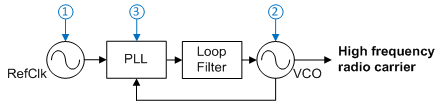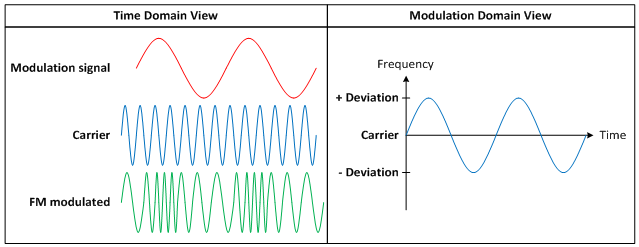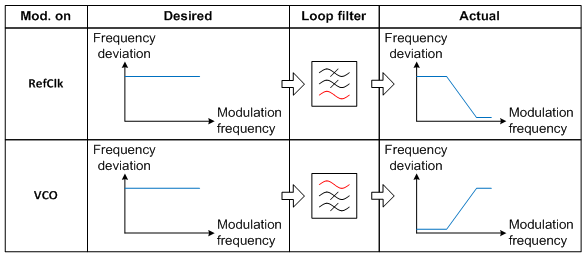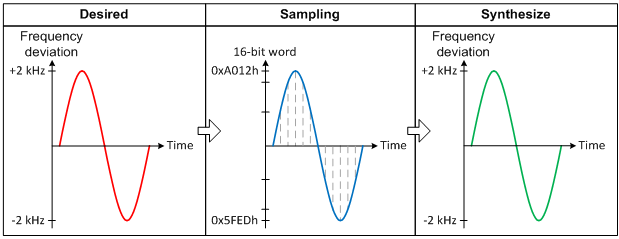# How to implement FM modulation

For most people, listening to frequency modulation (FM) radio is a part of their life. But what is FM exactly? It’s simply a popular modulation scheme used to embed information on a high-frequency radio carrier. The hardware requirements for implementing FM are low. Nevertheless, there are a couple of FM implementation techniques, each with different characteristics.

FM implementation

In general, you can implement FM in either an analog or digital way. Regardless of technique, you will need a frequency synthesizer (Figure 1) to generate the high-frequency radio carrier.Figure 1: Frequency synthesizer simplified block diagram

A frequency synthesizer is a closed-loop system that consists of a clean and stable low-frequency reference clock (RefClk), a phase-locked loop (PLL) chip, a loop filter that defines the bandwidth of the closed loop and a high-frequency voltage-controlled oscillator (VCO). The VCO will track the RefClk. Its frequency is equal to N x RefClk, where N is a number greater than or equal to 1. The loop filter response is low-pass to the RefClk and the PLL. That is, the RefClk and PLL outputs will be low-pass-filtered before going to the VCO. The loop filter response to the VCO, however, is high-pass-filtered.

Depending on the FM implementation technique, the modulation signal (the information that you want to embed in the high-frequency carrier) is applied to modulate the RefClk, VCO or PLL. The result of the modulation is that the carrier frequency will shift continuously. The amount of frequency shift is called frequency deviation. Figure 2 depicts a FM signal in time domain and modulation domain.Figure 2: FM modulation

FM implementation – analog technique

In the analog approach, the modulation signal is applied to either the RefClk or VCO. The advantages of this method are that the hardware is very simple and easy to implement. The drawbacks of this method are that performance is not consistent, or requires special care to make it consistent across different VCOs and modulation frequencies.

For example, let’s assume that RefClk = 20MHz and VCO = 480MHz. Since VCO = N x RefClk, N = 24. If a 1Vpp modulation signal is applied to the RefClk and produces a ±100Hz frequency deviation at the output of the RefClk, then the frequency deviation at the output of the VCO becomes ±2.4kHz. To achieve the same VCO frequency deviation for VCO = 960MHz, you must adjust the strength of the modulation signal, as N has become 48. Unfortunately, this adjustment may not necessarily be linear. In other words, a 0.5Vpp modulation signal may not return a ±50Hz frequency deviation. This is true even if the modulation signal is applied to the VCO. The VCO gain (Kvco) changes over the VCO frequency. Kvco refers to how the VCO frequency would change vs. the control voltage. If Kvco is not linear across the entire VCO operation range, then the same modulation signal strength applied to the VCO will result in different frequency deviations when the VCO frequency changes.

Furthermore, the loop bandwidth (LBW) will determine the usable modulation frequency. If the modulation is applied to the RefClk, the maximum modulation frequency will be less than the LBW because the loop filter is low-pass-filtered to the RefClk. In fact, the minimum modulation frequency has to be greater than the LBW if the modulation is applied to the VCO. In some use cases requiring a flat response, the modulation signal will be applied to both the RefClk and VCO simultaneously. Figure 3 shows the loop filter response to the RefClk and VCO.Figure 3: Analog implementation technique

FM implementation – digital technique

You can overcome the shortcomings of the analog technique by digitally synthesizing the FM modulation through the PLL. If VCO = N x RefClk, by continuously changing the N value in a precise manner, you can synthesize the modulation domain view waveform, as shown in Figure 2. The advantages of this method are that the frequency deviation does not depend on Kvco and the VCO frequency anymore. In addition, if the LBW is wide enough, the modulation frequency response will be flat. The trade-off is that this method requires higher digital processing power from the logics because the PLL requires continuous programming. Plus, the synthesizing rate (or sampling rate) must be higher than the modulation frequency in order to reduce the sidebands created from sampling. For more details on this method, see the application report, “Frequency Shift Keying with LMX2571.” Figure 4 shows how the desired waveform is sampled and synthesized with LMX2571.Figure 4: Digital implementation technique

Which technique is better?

In analog FM radio broadcasting system, the analog technique will be better. It is because the carrier is usually fixed at a particular frequency. The shortcomings of this technique do not happen at all.

In applications with multiple carrier channels, for example, 2-way radio, the digital technique will be superior to the analog technique.

In general, both techniques have their pros and cons. The adoption will depend on the application needs, system capability, performance index, and cost.